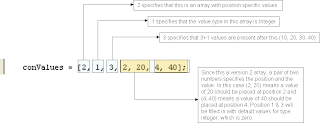### Create() method in the AX foundation classes.

When it comes to creating and using Maps, Lists or Arrays, we normally initialize them using the new() method. Then we use the respective methods on these to insert the data. All these classes have a static method create() which can both initialize and insert values into these at the same time.Even though, the method takes a container parameter in all the three cases, the content of the containers are very different from each other.

Let us look at all the three in detail. Download the XPO for the source code from here.

Code for Array class.

`static void ArrayInit(Args _args){    Array       ageArray,                nameArray;    container   conValues;    counter     i;    ;        conValues = [1, 1, 3, 10, 20, 30, 40];    ageArray = Array::create(conValues);    print "Printing age array (consecutive values) ...";    for(i=1; i <= ageArray.lastIndex(); i++)    {        print ageArray.value(i);    }        conValues = [2, 1, 3, 2, 20, 4, 40];    ageArray = Array::create(conValues);    print "Printing age array  (position specific values)...";    for(i=1; i <= ageArray.lastIndex(); i++)    {        print ageArray.value(i);    }        conValues = [1, 0, 4, "Aaron", "Billy", "Clint", "Danny", "Elmer"];    nameArray = Array::create(conValues);    print "Printing name array ...";    for(i=1; i <= nameArray.lastIndex(); i++)    {        print nameArray.value(i);    }    pause;}`

The container should be packed with the following values

Version of the Array – this can take either 1 or 2. 1 is for consecutive value arrays and 2 for position specific arrays. More detail on this will come shortly.

Value type – Specify the type of the values that are being inserted into the array. To know the value for each type, check this entry

Number of values – This is a zero based values which specifies how many values are defined after the Number of values argument. Suppose you are providing 4 values, this parameter should be then 3 since this is zero based.

In the above example, look at the ageArray container. The values we pack into the container are described below clearly.Let us look at the second initialization of ageArray container. This time the version is 2Code for List creation.
`static void ListInit(Args _args){    List            ageList;    ListEnumerator  enumerator;    container       conValues;    counter         i;    ;    conValues = [1, 1, 3, 100, 200, 300];    ageList = List::create(conValues);    enumerator = ageList.getEnumerator();    while(enumerator.moveNext())    {        print enumerator.current();    }    pause;}`

The values to be packed into the container for the List class are

Version of the list – This specifies the version of the list. This is always 1

Value type – Specify the value type of the values that are being inserted into the array.

Number of values – Specifies the number of values being added into the list. This is not zero based.

Code for Map creation
`static void MapInit_(Args _args){    Map             ageMap;    MapEnumerator   enumerator;    container       conValues;    counter         i;    ;    conValues = [1, 1, 1, 3, 1, 100, 2, 200, 3, 300];    ageMap = Map::create(conValues);    enumerator = ageMap.getEnumerator();    while(enumerator.moveNext())    {        print enumerator.current();    }    pause;}`

The values to be packed into the container for the Map class are

Version of the map – This specifies the version of the map. This is always 1

Key type – Specify the type of the keys that are being inserted into the map.

Value type – Specify the type of the values that are being inserted into the map.

Number of key, value pairs – Specifies the number of values being added into the map. This is not zero based.

The values should be entered in pairs.

conValues = [1, 1, 1, 3, 1, 100, 2, 200, 3, 300];

Here, 1 is the map version, 1, 1 specifies that key & value type are integer. 3 specifies that there are 3 pairs, (1, 100), (2, 200) & (3, 300) are the three key value pairs.

I hope this post was helpful in understanding the create method that is present on these classes.